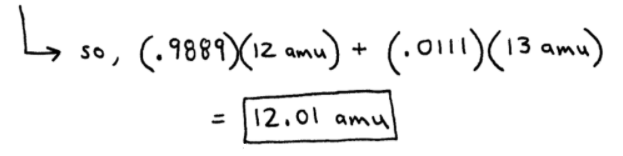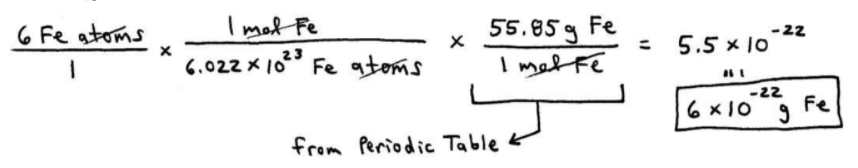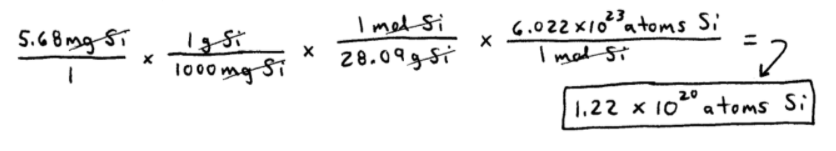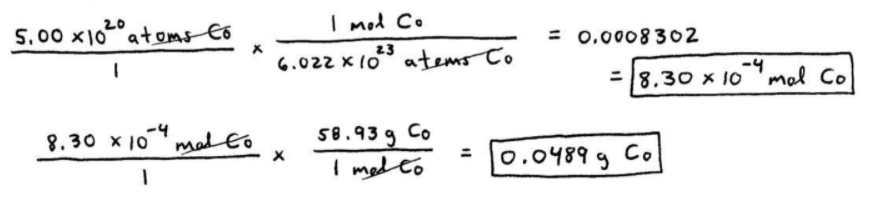# S3E1 - Avogadro's Number, and Grams to Moles Calculations

## Atomic Mass and "AMU"

Atomic Masses  -  In 1961 a system was devised to assign atomic masses to each element.

To do this, a "standard" was needed...

An isotope of carbon, carbon-12 (12C), was used as the standard and was assigned a value of exactly 12 amu.

➞  amu  =  atomic mass units

==========

## Atomic Mass and Isotopes

Question Why is carbon's atomic mass equal to 12.01 amu on the Periodic Table
_________

➞  most elements occur as a mixture of isotopes and natural carbon is found as 98.89% 12C and 1.11% 13C atoms:As you can see in the graphic above, atomic mass is a "weighted average" of isotopes.

Notice that not a single carbon atom weighs 12.01 amu!!  This is an average weight.

The math involved in calculating "weighted averages" is why atomic masses of elements are expressed as decimals on the Periodic Table.

----------

ex:  Lithium (Li)  =  6.941amu

==========

## The Mole and Avogadro's Number

The Mole  =  1 mole of any substance contains exactly 6.022 x 1023 items of that substance.

The mole is often abbreviated as "mol."

examples:

"1 dozen eggs"  =  12 eggs
"1 mol of eggs"  =  6.022 x 1023 eggs

examples:

1 mole Fe atoms  =  6.022 x 1023 atoms of Fe
1 mole bananas  =  6.022 x 1023 bananas
1 mole H2O molecules = 6.022 x 1023 molecules H2O

### 6.022 x 1023 is Avogadro's Number (NA)

----------

** The mass (g) of 1 mole of an element is equal to its atomic mass (amu).

➞  So for carbon...

12.01 amu = 12.01 grams per mole = 12.01 g/mol

And this is true for every element in the Periodic Table.

==========

## Atoms to Grams Calculation:

ex:  Find the mass in grams of a sample of 6 Fe atoms.

Remember?...==========

## Grams to Moles Calculation:

ex:  Find both the number of moles of atoms and the actual number of atoms in a 10.0 g sample of aluminum.And to calculate the number of aluminum atoms, we have:==========

## Grams to Atoms Calculation:

ex:  A silicon chip has a mass of 5.68 mg. How many silicon atoms are present?## Atoms to Moles, Atoms to Grams Calculations:

ex:  Calculate the number of moles and the mass of a sample of cobalt containing 5.00 x 1020 atoms of Co.==========

In my next post on SECTION 3 - Chemical Quantities and Stoichiometry,